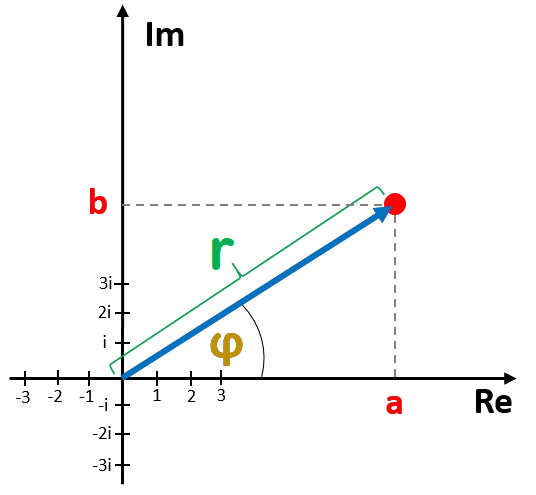# Complex Number to Rectangular Form Calculator

Created by Anna Szczepanek, PhD
Reviewed by Komal Rafay
Last updated: May 04, 2023

Omni's complex number to rectangular form calculator will help you whenever you're stuck with a complex number in its polar form (the one with exponent, modulus, and argument) and need it quickly converted from this exponential form to rectangular form.

In what follows, we will recall what the rectangular form is all about and what formulas stand behind the conversion from polar to rectangular form, so that, in case of emergency, you know how to find rectangular form of complex number by hand.

## What is the rectangular form of a complex number?

The rectangular form is the most common way of writing down complex numbers as z = a + bi. It corresponds to representing z as a point (a, b) on a 2D complex plane. It means that z is a number with a units along the real (horizontal) axis and b units along the imaginary (vertical) axis.

The two coordinates have their names:

• a is the real part of z; and
• b is the imaginary part of z.In the next section we will explain how to convert a complex number from polar to rectangular form.

## Conversion of a polar complex number to rectangular form

If we have to convert a complex number z = r × exp(iφ) from its polar (exponential) form to rectangular form, we must recall the basic trigonometric formulas:

cos(φ) = a / r

and

sin(φ) = b / r.

Solving for a and b, respectively, we obtain

a = r × cos(φ)

and

b = r × sin(φ)

These are the formulae that we use to convert from polar form to rectangular form. They are implemented in Omni's complex number to rectangular form calculator as well.

## How to use this complex number to rectangular form calculator

This tool is very straightforward to use. If you want to convert polar complex number to rectangular form, input the two ingredients of the polar form: the magnitude and phase into the two upper fields of the calculator. It will immediately display the two coefficients of the rectangular form of your number: the real part a and the imaginary part b. You write them down in the a + bi form.

🙋 Note that while inputting the phase (argument) of your number into our complex number to rectangular form calculator you can freely choose between radians and degrees.

That's it! Never again will you have to wonder how to find rectangular form of complex number :)

## Complex numbers with Omni calculators

Once you're done with converting polar complex numbers to rectangular form, learn how to convert to other forms as well! Omni has a rich collection of calculators that will help you master various operations on complex numbers:

## FAQ

### How do I find the rectangular form of a complex number?

To convert a polar number z = r × exp(iφ) to rectangular form:

1. Compute cos(φ).
2. Multiply by r. This is the real part of your number: a = r × cos(φ).
3. Compute sin(φ) .
4. Multiply by r.
5. This is the imaginary part of your number b = r × sin(φ).
6. Your number in rectangular form reads z = a + bi with a, b computed as above.

### What is the rectangular form of exp(iπ/3)?

The answer is 1/2 + (√3/2)i. To arrive at this result, we compute cos(π/3) = 1/2 and sin(π/3) = √3/2. The magnitude of exp(iπ/3) is 1. So:

• The real part is a = 1 × cos(π/3) = 1 × 1/2 = 1/2.
• The imaginary part is b = 1 × cos(π/3) = 1 × √3/2 = √3/2.
Anna Szczepanek, PhD
Polar form r × exp(iφ)
Magnitude (r)
Phase (φ)
deg
Rectangular form a + bi
Real part (a)
Imaginary part (b)
People also viewed…

Addiction calculator tells you how much shorter your life would be if you were addicted to alcohol, cigarettes, cocaine, methamphetamine, methadone, or heroin.

### Body fat

Use our free Body Fat Calculator, based on BMI, to determine your body fat percentage and explore your ideal body fat range.

### Condition number

The condition number calculator finds the condition number of a matrix. It supports the use of popular matrix norms and can find a 2×2 or 3×3 matrix's condition number.

### Sine

Use our sin calculator to find out the sine value for chosen angle.